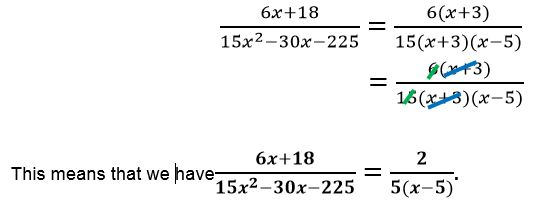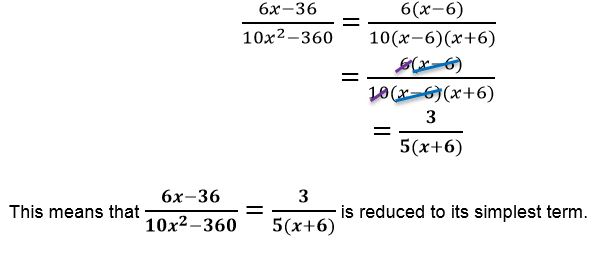# How to simplify algebraic fractions

Want to find out how to simplify algebraic fractions? We've provided a step-by-step guide of how to simplify algebraic fractions to teach you everything you need to know.## Step 1: Find the common factors

Look for the factors shared by the numerator’s coefficients and do the same for the factors shared by the denominator’s coefficients. For example, if we want to simplify (5x^2-125)/(20x+100), we have Common factors shared by 5 and 125 → 5 Common factors shared by 20 and 100 →20

## Step 2: Factor out the remaining algebraic expression

Factor out the remaining algebraic expression left on the numerator and denominator completely. For our example, the denominator is already simplified. We can apply the difference of two squares property: a^2-b^2=(a-b)(a+b).

## Step 3: Cancel common factors

Cancel common factors shared by the numerator and denominator.

## Examples of how to simplify algebraic fractions

Q1) Simplify (6x+18)/(15x^2-30x-225) to its lowest terms.

Find the common factors shared by the coefficients of 6x+18 and factors shared by the coefficients of 15x^2-30x-225. 6x+18=6(x+3) 15x^2-30x-225=15(x^2-2x-15)

Factor out the remaining algebraic expressions found in the numerator and denominator. Since 6(x+3) is already completely factored out. We can then focus on x^2-2x-15.

x^2-2x-15=(x+3)(x-5)

Express the numerator and denominator as in their completely factored out forms and cancel out the common factors:Q2) What would be the expression if we reduce (6x-36)/(10x^2-360) to its simplest form?

Determine the common factors shared by the coefficients of 6x-36 as well as 10x^2-360.

6x-36=6(x-6) 10x^2-360=10(x^2-36)

Once the greatest common factor is factored out of the expressions, we can factor out the algebraic expressions completely.

2x-1 is already in its simplest form so, we can focus on factoring our x^2-36 completely using the property: a^2-b^2=(a-b)(a+b)

x^2-36=(x)^2-(6)^2 =(x-6)(x+6)

Express the numerators and denominators in their factored out form and cancel out common factors.#### Join today

The fastest way to practice

Unlock our complete testing platform and improve faster that ever.